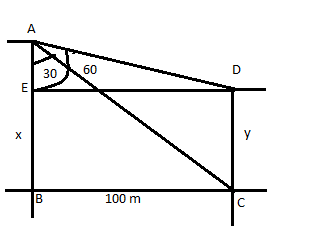# SSC CGL 2018 Practice Test Papers | Quantitative Aptitude (Day-7)

Dear Aspirants, Here we have given the Important SSC CGL Exam 2018 Practice Test Papers. Candidates those who are preparing for SSC CGL 2018 can practice these questions to get more confidence to Crack SSC CGL 2018 Examination.

[WpProQuiz 2721]

Click “Start Quiz” to attend these Questions and view Explanation

1. If x= 1/7 y and y= 1/3 z then what is the ratio of x:z?
1. 2:1
2. 3: 1
3. 1:21
4. 21:1
1. The ratio of two numbers is 7: 5 and the product of two numbers is 7875. What is the difference between the two numbers?
1. 30
2. 60
3. 20
4. 50
1. The average monthly income of 3 members of a family is 20000. The salary of one of the member of the family is 10000 and the difference of salary between other 2 members is 16000 then what is the highest income of the family?
1. 33000
2. 30000
3. 40000
4. 50000
1. A and B can do a piece of work in 12 days. With the help of C and D the work was completed in 6 days. A is three times efficient as C and A can do the work in 20 days. Find the time taken by D to complete the work?
1. 20
2. 30
3. 60
4. 15
1. 64 80 120 ? 420
1. 240
2. 230
3. 300
4. 210
1. In an electrical appliance shop, the sales is illustrated in a diagram. Observe the figure and answer the following questions. Circle represents sales of fan, Triangle represents sales of fridge and Rectangle represents sales of TV. Find the number of persons who bought both TV and Fridge.
1. 10
2. 17
3. 20
4. 5

7.In an electrical appliance shop, the sales is illustrated in a diagram. Observe the figure and answer the following questions. Circle represents sales of fan, Triangle represents sales of fridge and Rectangle represents sales of TV. What is the difference between sales of Tv and fan.

1. 0
2. 7
3. 10
4. 5
1. In an electrical appliance shop, the sales is illustrated in a diagram. Observe the figure and answer the following questions. Circle represents sales of fan, Triangle represents sales of fridge and Rectangle represents sales of TV. Find the number of sales of all the three appliances.
1. 10
2. 5
3. 7
4. 21
1. The angle of depression of top and bottom of a tree from a building is 60o and 30o respectively. The distance between the tree and the building is 100 m. What is the height of the building?
1. 100√3 m
2. 100(√3-1) m
3. 100 m
4. 200 m
1. The value of 4/5+ 16 3/5 x27 is
1. 430
2. 449
3. 512
4. 345

X:y=1:7

Y:z=1:3

X   :   y:  z

1   :  7

1:    3

1:     7:     21=>x: y: z

Therefore, x:z= 1:21

Let the two numbers be 7x and 5x respectively.

7x*5x=7875

X2=225=> x=15

Difference of two numbers= 2*15=30

Let the salaries be x, y and z respectively.

Sum of income of 3 members= 20000×3=60000

X=10000

y-z=16000

x+y+z=60000

On solving y=33000, z=17000

A+B=1/12

A+B+C+D=1/6

A=1/20=>C=1/60

A+B+C=1/12+1/60=1/10

D=A+B+C+D-(A+B+C) = 1/6-1/10=1/15

D alone can complete the work in 15 days.

x5/4, x6/4, x7/4, x8/4 series

10+7=17

Sale of fan= 3+32+10+5= 50

Sale of TV=  10+7+5+18=40

Difference= 50-40=10

Explanation:In         ABC,

tan 30o=BC/AB

1/√3=100/AB

AB=100√3 m